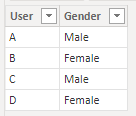cancel
Showing results for
Did you mean:Helper I

## How to count Average of Text?

Hi i have a data column Gender

I need to count Male and Female and the average percentage will be 50%

1 ACCEPTED SOLUTIONCommunity Support

Hi @blockobito ,

If you want to calculate the percentage of males and females in the total, you can try this code.

``````Percentage =
VAR _Total =
CALCULATE ( COUNT ( 'Table'[User] ), ALL ( 'Table' ) )
VAR _Each_Gender =
CALCULATE ( COUNT ( 'Table'[User] ) )
RETURN
DIVIDE ( _Each_Gender, _Total )``````

My Sample:Result is as below.Best Regards,
Rico Zhou

If this post helps, then please consider Accept it as the solution to help the other members find it more quickly.

2 REPLIES 2Community Support

Hi @blockobito ,

If you want to calculate the percentage of males and females in the total, you can try this code.

``````Percentage =
VAR _Total =
CALCULATE ( COUNT ( 'Table'[User] ), ALL ( 'Table' ) )
VAR _Each_Gender =
CALCULATE ( COUNT ( 'Table'[User] ) )
RETURN
DIVIDE ( _Each_Gender, _Total )``````

My Sample:Result is as below.Best Regards,
Rico Zhou

If this post helps, then please consider Accept it as the solution to help the other members find it more quickly.Helper I

Measure = CountRows(table)

Then place a visual with the gender and the Measure as the column. Can be added twice and use second as % of total.

If you need to split it up into separate records...

Measure Male =

CALCULATE(COUNTROWS(Table1),Table1[gender] = "Male")
(you will need to ensure that the data is all uniform ie all CAPS or all lower etc I think)